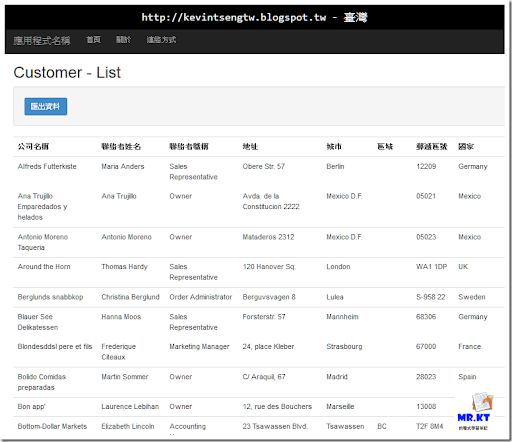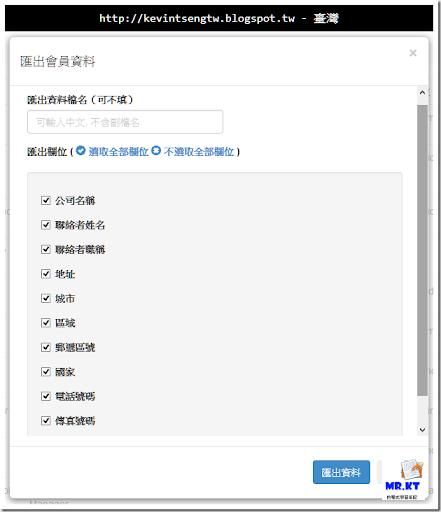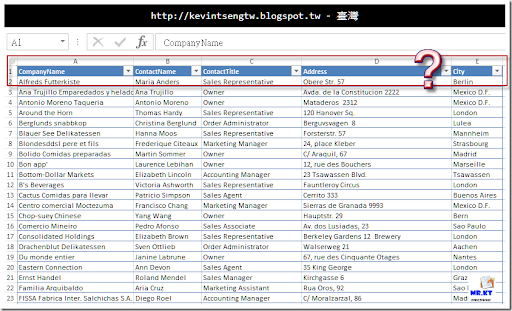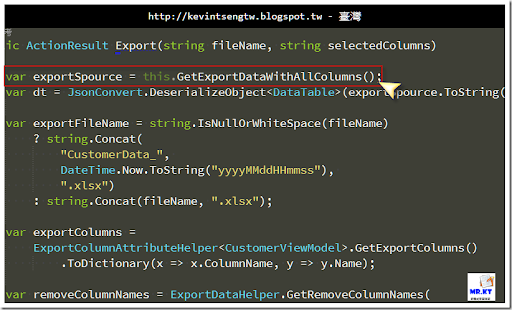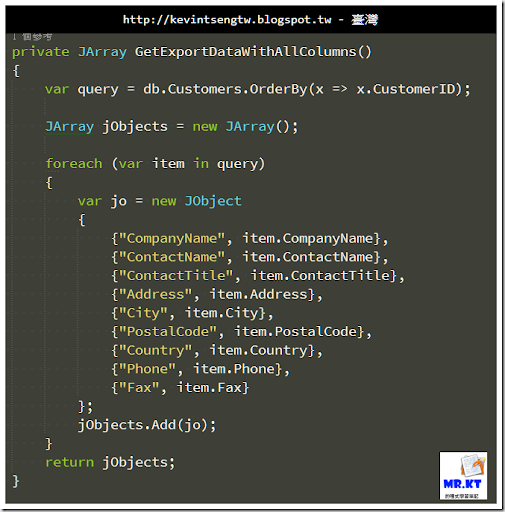## 2014年10月5日 星期日

### ASP.NET MVC 匯出 Excel - 讓使用者挑選要匯出的資料欄位 Part.2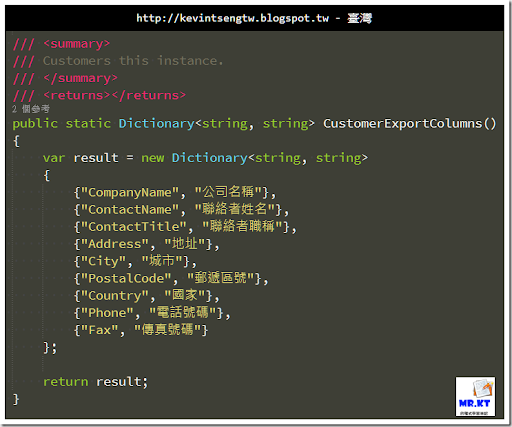ExportColumnAttributes.cs

`[System.AttributeUsage(System.AttributeTargets.Property, AllowMultiple = false)]`
`public class ExportColumnAttribute : Attribute`
`{`
`    /// <summary>`
`    /// 欄位顯示名稱.`
`    /// </summary>`
`    /// <value>`
`    /// The name.`
`    /// </value>`
`    public string Name { get; set; }`
` `
`    /// <summary>`
`    /// 欄位顯示順序.`
`    /// </summary>`
`    /// <value>`
`    /// The order.`
`    /// </value>`
`    public int Order { get; set; }`
` `
`    public ExportColumnAttribute()`
`    {`
`    }`
`}`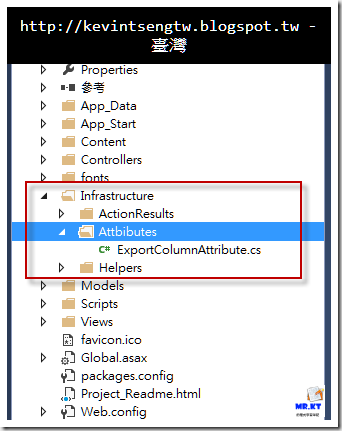ExportColumnAttributeHelper.cs

`public class ExportColumnAttributeHelper<T> where T : class`
`{`
`    /// <summary>`
`    /// Gets the export columns.`
`    /// </summary>`
`    /// <returns></returns>`
`    public static List<ExportColumnObject> GetExportColumns()`
`    {`
`        var type = typeof(T);`
`        var infos = type.GetProperties();`
` `
`        var result = infos.Select(x => x.Name)`
`                          .Select(propertyName => GetProperty(type, propertyName))`
`                          .Select(GetExportColumnInstance)`
`                          .Where(instance => instance != null)`
`                          .ToList();`
` `
`        return result.OrderBy(x => x.Order).ToList();`
`    }`
` `
`    /// <summary>`
`    /// Gets the export column instance.`
`    /// </summary>`
`    /// <param name="pInfo">The p information.</param>`
`    /// <returns></returns>`
`    public static ExportColumnObject GetExportColumnInstance(PropertyInfo pInfo)`
`    {`
`        if (null == pInfo) return null;`
`        try`
`        {`
`            var arr = pInfo.GetCustomAttributes(typeof(ExportColumnAttribute), true);`
` `
`            if (arr.Length.Equals(0)) return null;`
` `
`            var attr = arr as ExportColumnAttribute;`
` `
`            var instance = new ExportColumnObject()`
`            {`
`                ColumnName = pInfo.Name,`
`                Name = attr.Name,`
`                Order = attr.Order`
`            };`
` `
`            return instance;`
`        }`
`        catch`
`        {`
`            return null;`
`        }`
`    }`
` `
`    /// <summary>`
`    /// Gets the property.`
`    /// </summary>`
`    /// <param name="type">The type.</param>`
`    /// <param name="propName">Name of the property.</param>`
`    /// <returns></returns>`
`    private static PropertyInfo GetProperty(Type type, string propName)`
`    {`
`        try`
`        {`
`            var infos = type.GetProperties()`
`                            .Where(info => propName.ToLower().Equals(info.Name.ToLower()));`
` `
`            foreach (var info in infos)`
`            {`
`                return info;`
`            }`
`        }`
`        catch`
`        {`
`            throw;`
`        }`
`        return null;`
`    }`
`}`
`ExportColumnObject.cs`
`public class ExportColumnObject`
`{`
`    public Guid ID { get; set; }`
` `
`    /// <summary>`
`    /// 欄位輸出名稱.`
`    /// </summary>`
`    /// <value>`
`    /// The name.`
`    /// </value>`
`    public string Name { get; set; }`
` `
`    /// <summary>`
`    /// 欄位順序.`
`    /// </summary>`
`    /// <value>`
`    /// The order.`
`    /// </value>`
`    public int Order { get; set; }`
` `
`    /// <summary>`
`    /// 欄位（屬性）名稱.`
`    /// </summary>`
`    /// <value>`
`    /// The name of the column.`
`    /// </value>`
`    public string ColumnName { get; set; }`
` `
`    public ExportColumnObject()`
`    {`
`        this.ID = Guid.NewGuid();`
`    }`
` `
`}`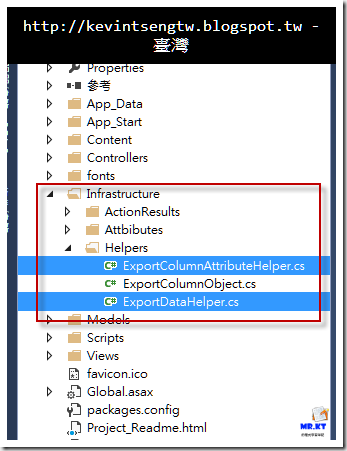ViewModel 類別是用於 View 的顯示之用，單純只有屬性、欄位而沒有任何的行為、方法，而且有時候我們所要匯出的資料並不是從單一個類別而來，會是從各個類別所來的資料組合而成，而匯出資料的處理是經過多個操作與彙整，所以我們要看待的並不是原生的模型類別，而是最後匯出資料的類別。

CustomerViewModel.cs

`public class CustomerViewModel`
`{`
`    [Display(Name = "Customer ID")]`
`    public string CustomerID { get; set; }`
` `
`    [Display(Name = "公司名稱")]`
`    [ExportColumn(Name = "公司名稱", Order = 1)]`
`    public string CompanyName { get; set; }`
` `
`    [Display(Name = "聯絡者姓名")]`
`    [ExportColumn(Name = "聯絡者姓名", Order = 2)]`
`    public string ContactName { get; set; }`
` `
`    [Display(Name = "聯絡者職稱")]`
`    [ExportColumn(Name = "聯絡者職稱", Order = 3)]`
`    public string ContactTitle { get; set; }`
` `
`    [Display(Name = "地址")]`
`    [ExportColumn(Name = "地址", Order = 4)]`
`    public string Address { get; set; }`
` `
`    [Display(Name = "城市")]`
`    [ExportColumn(Name = "城市", Order = 5)]`
`    public string City { get; set; }`
` `
`    [Display(Name = "區域")]`
`    [ExportColumn(Name = "區域", Order = 6)]`
`    public string Region { get; set; }`
` `
`    [Display(Name = "郵遞區號")]`
`    [ExportColumn(Name = "郵遞區號", Order = 7)]`
`    public string PostalCode { get; set; }`
` `
`    [Display(Name = "國家")]`
`    [ExportColumn(Name = "國家", Order = 8)]`
`    public string Country { get; set; }`
` `
`    [Display(Name = "電話號碼")]`
`    [ExportColumn(Name = "電話號碼", Order = 9)]`
`    public string Phone { get; set; }`
` `
`    [Display(Name = "傳真號碼")]`
`    [ExportColumn(Name = "傳真號碼", Order = 10)]`
`    public string Fax { get; set; }`
`}`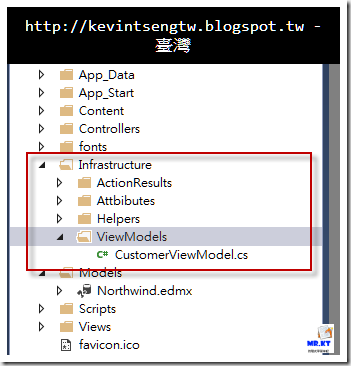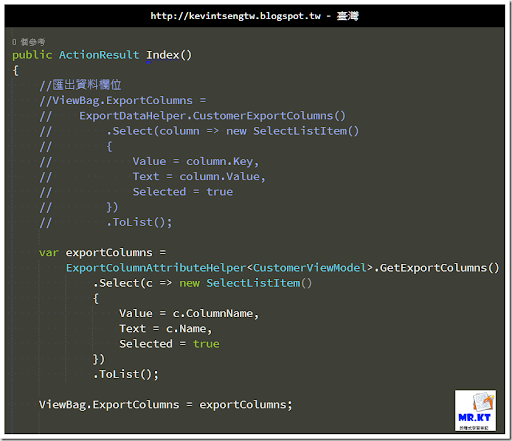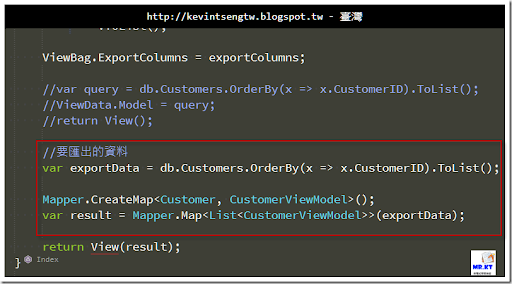~/Views/Customer/Index.cshtml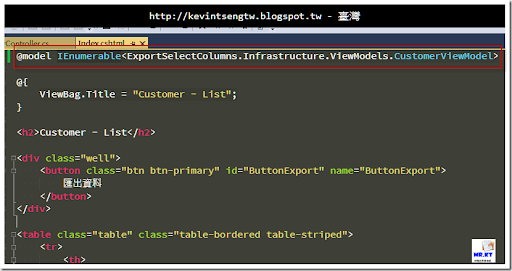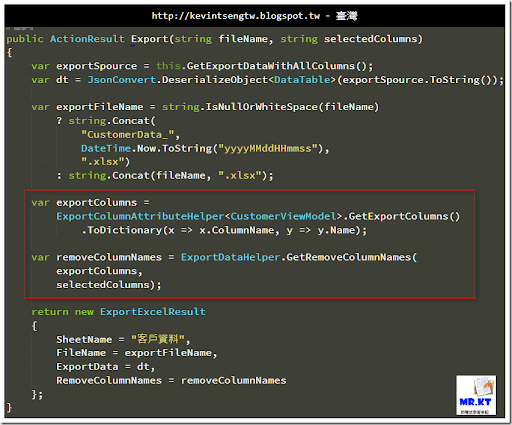### 執行結果